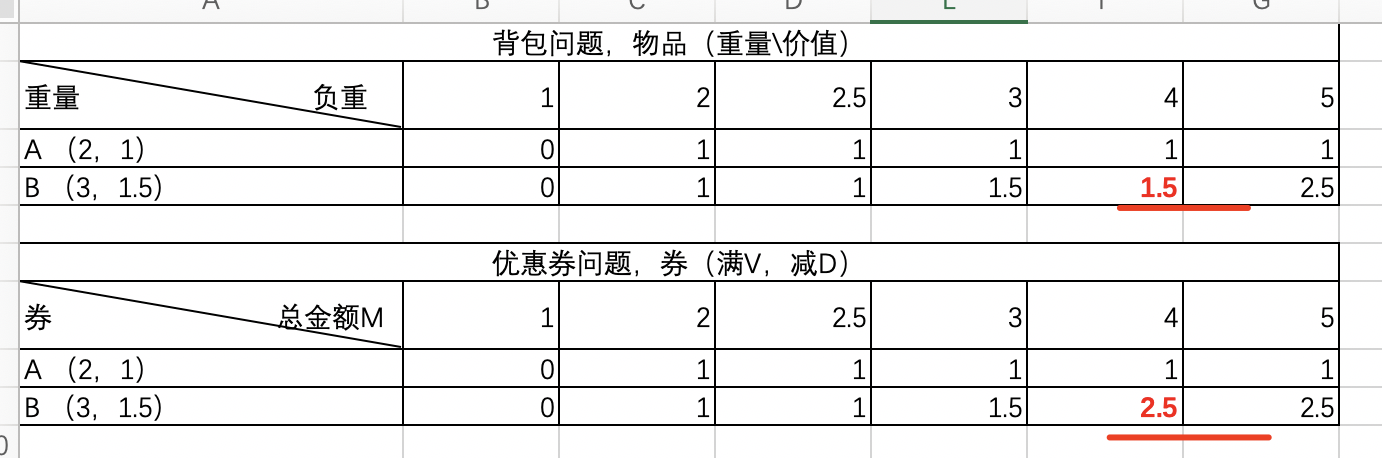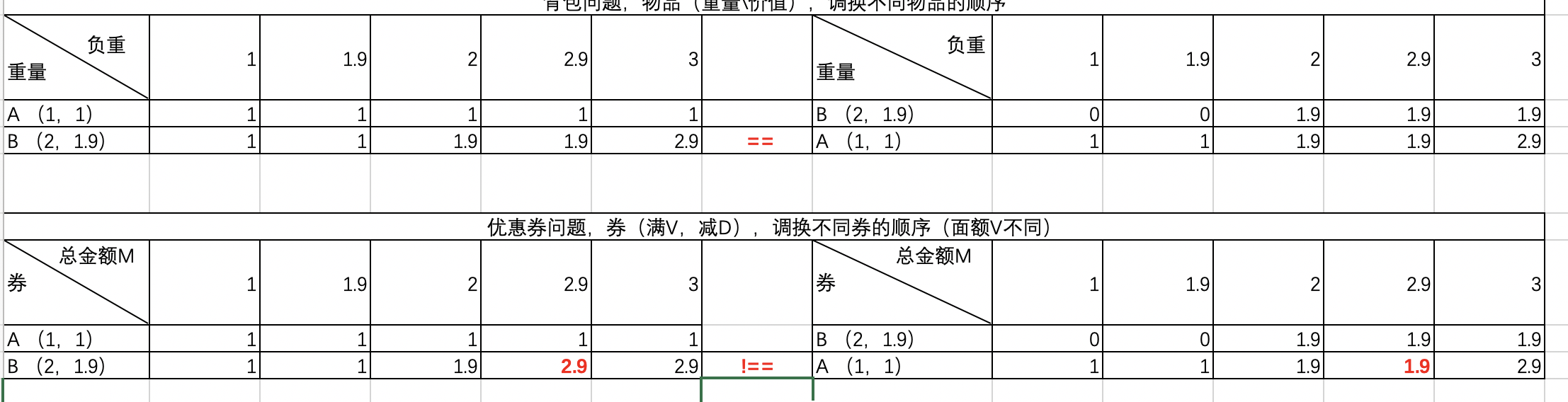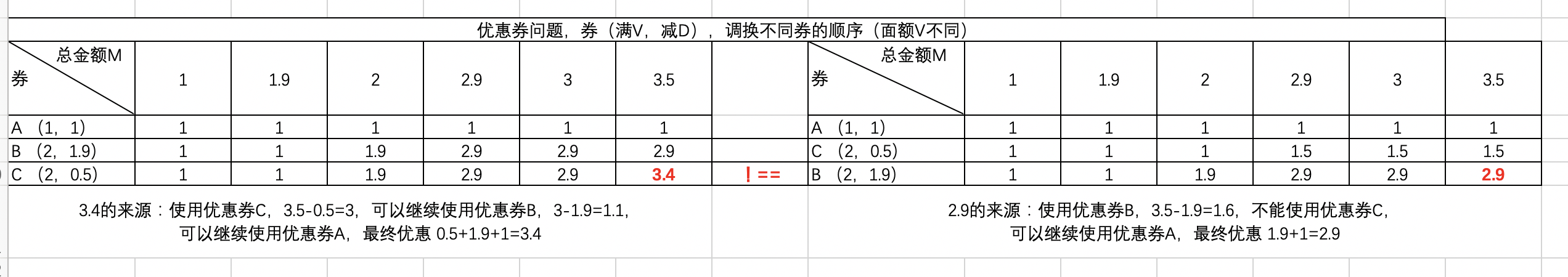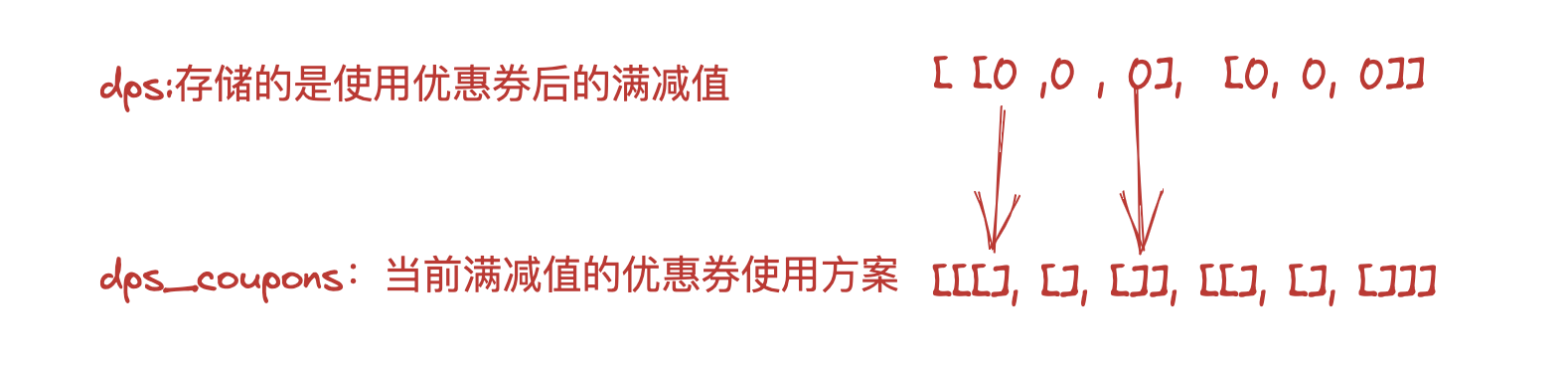# 01、关于满减优惠券可叠加使用场景下的动态规划算法

## 01、关于满减优惠券可叠加使用场景下的动态规划算法

### 1、需求简介

设共有n张优惠券C: [(V1, D1), (V2, D2), (V3, D3), ..., (Vn, Dn)]，其中Vn为面值，Dn为减免值（对于一张优惠券Cx，满Vx减Dx），优惠券为单张，可叠加使用（使用过一张后，如果满足面值还可以使用其他优惠券）。



C = [(2, 1.9), (1, 1), (1, 0.1), (2, 0.1)] , M = 3

### 2、优惠券问题和背包问题的不同点• 背包判断大于重量W，再减去W，得到剩余值，根据剩余值再去上一层找剩余值对应的值（统计价值），背包问题是减去重量W
• 优惠券则是当总金额M大于面额V时，再减去减免值D，得到剩余的金额N，再根据金额N去上一层找对应的值（统计减免值D），优惠券问题是减去减免值D

### 3、优惠券的顺序问题

#### 3.1 对于优惠券，不同面额的顺序#### 3.2 面额相同，减免值不同[
(2, 1.9),
(1, 1),
(1, 0.1),
(2, 0.1)
]


[
(1, 1),
(1, 0.1),
(2, 1.9),
(2, 0.1),
]


### 4、程序实现

#### 4.1 具有稳定性的排序算法

def merge(nums: list[int], left: int, mid: int, right: int) -> None:
""" 合并左子数组和右子数组 """
# 左子数组区间 [left, mid]
# 右子数组区间 [mid + 1, right]
# 初始化辅助数组
tmp: list[int] = list(nums[left:right + 1])
# 左子数组的起始索引和结束索引
left_start: int = 0
left_end: int = mid - left
# 右子数组的起始索引和结束索引
right_start: int = mid + 1 - left
right_end: int = right - left
# i, j 分别指向左子数组、右子数组的首元素
i: int = left_start
j: int = right_start
# 通过覆盖原数组 nums 来合并左子数组和右子数组
for k in range(left, right + 1):
# 若“左子数组已全部合并完”，则选取右子数组元素，并且 j++
if i > left_end:
nums[k] = tmp[j]
j += 1
# 否则，若“右子数组已全部合并完”或“左子数组元素 <= 右子数组元素”，则选取左子数组元素，并且 i++
elif j > right_end or tmp[i] <= tmp[j]:
nums[k] = tmp[i]
i += 1
# 否则，若“左右子数组都未全部合并完”且“左子数组元素 > 右子数组元素”，则选取右子数组元素，并且 j++
else:
nums[k] = tmp[j]
j += 1

def merge_sort(nums: list[int], left: int, right: int) -> None:
""" 归并排序 """
# 终止条件
if left >= right:
return                        # 当子数组长度为 1 时终止递归
# 划分阶段
mid: int = (left + right) // 2    # 计算中点
merge_sort(nums, left, mid)       # 递归左子数组
merge_sort(nums, mid + 1, right)  # 递归右子数组
# 合并阶段
merge(nums, left, mid, right)

""" Driver Code """
if __name__ == '__main__':
nums: list[int] = [ 7, 3, 2, 6, 0, 1, 5, 4 ]
merge_sort(nums, 0, len(nums) - 1)
print("归并排序完成后 nums =", nums)


#### 4.2 优惠券叠加算法

# coding:utf-8
# 背包算法，解决满减优惠券叠加使用问题

def coupon_bags(coupon, amount):
"""
优惠券背包算法
param: coupon 优惠券数组
param: amount 金额
"""
# 转换金额跨度（间隔）： 元->角
span = 10
amount = int(amount*span)

for i, v in enumerate(coupon):
for j in range(len(v)):
coupon[i][j] = int(coupon[i][j]*span)

# 初始化结果数组，dps 存储满减值（背包算法结果）
# dps_coupons 存储 dps 对应满减值下使用的 优惠券方案
dps = []
dps_coupons = []
# len(coupon)+1，这里为什么要加 1，
# 记住 动态算法规划算法背包问题一文中的： dp[i] = dq[j]=0;原因就是这个
for i in range(len(coupon)+1):
# 这里为啥是 amount+1,因为 dps 中的索引是从0开始的，
# 索引0的位置处存的是金额0的满减值，所以这里需要 amount+1
dps.append(list((0,)*(amount+1)))

# list 直接 * 生成的是同一list，用循环生成
dps_coupons.append([])
for j in range(amount+1):
dps_coupons[i].append([])

for i in range(1, len(coupon)+1):
# i 代表第 i 张优惠券，从 1 开始的
for j in range(1, amount+1):
# j 代表的是 总金额M为：j
# coupon[i-1] 代表的是优惠券的中的 V，即满 coupon[i-1] 减 coupon[i-1]
if j < coupon[i-1]:  # 金额j 达不到 满减的条件：V，取上一层对应位置的值
# 获取上个策略值
dps[i][j] = dps[i-1][j]
dps_coupons[i][j] = dps_coupons[i-1][j]
else:  # 金额j 达到了 满减的条件：V

# dps中的i代表的是第几张优惠券，j代表的是总金额为J时的最优满减值
# coupon[i - 1]，代表的是：当前优惠券的减免值
# dps[i - 1][j - coupon[i - 1]]，代表的是剩余金额对应的最优满减值
if dps[i - 1][j] > coupon[i - 1] + dps[i - 1][j - coupon[i - 1]]:
# 上一行同列数据 优于 当前优惠券+剩余的金额对应的上次数据，取之前数据
dps[i][j] = dps[i-1][j]
dps_coupons[i][j] = dps_coupons[i-1][j]
else:
# 选取当前+剩余 优于 上一行数据
dps[i][j] = dps[i-1][j-coupon[i-1]]+coupon[i-1]
# dps_coupons[i-1][j-coupon[i-1]]，值是list，需要拷贝，才不会影响之前的结果
dps_coupons[i][j] = dps_coupons[i-1][j-coupon[i-1]].copy()
# 表示使用了第 i 张优惠券
dps_coupons[i][j].insert(0, list(coupon[i-1]))

# 结果需返回数据原单位（元）
result_coupons = dps_coupons[-1][-1].copy()
for i, v in enumerate(result_coupons):
for j in range(len(v)):
result_coupons[i][j] = result_coupons[i][j]/span
print(f"使用优惠券：{result_coupons} 总减免：{dps[-1][-1]/span}")

# 优惠券
coupon_items = [
[1, 1],
[1, 0.1],
[2, 1.9],
[2, 0.1],
]
# 举例中的优惠券是最终顺序。确保优惠券已经排序过，多维升序(V升D降)，此处省略
coupon_bags(coupon_items, 3)
"""
coupon_items = [
[1, 0.6],
[2, 0.7],
[2, 1.3],
[3, 2.3],
]
coupon_bags(coupon_items, 5)
"""dps,dps_coupons数据存储示意图

#### 4.3 停车场优惠券叠加使用的场景

class CouponSort(object):
"""
具有稳定性的归并排序
coupon_list: [[5, 1, 189], [6, 1, 200]]
[5, 1, 189] --> 5: 总金额为5，1：减免值，189：此张优惠券对应数据库中的记录ID
"""
def __init__(self, coupon_list):
tmp = coupon_list.copy()
self.coupon_list = tmp
self.length = len(coupon_list)

def sort(self):
left = 0
right = self.length
self._merge_sort(left, right - 1, True)

# 下面是当总金额M相同时，按照减免值降序排序
start, end, index = 0, 0, 0
while index != self.length:
if index != self.length - 1:
if self.coupon_list[index] != self.coupon_list[index + 1]:
self._merge_sort(start, end, False)
start = index + 1
end = start
else:
end += 1
else:
self._merge_sort(start, end, False)
index += 1

return self.coupon_list

def _merge_sort(self, left, right, l2m):
""" 归并排序 """
# 终止条件
if left >= right:
return  # 当子数组长度为 1 时终止递归
# 划分阶段
mid = (left + right) // 2  # 计算中点
self._merge_sort(left, mid, l2m)  # 递归左子数组
self._merge_sort(mid + 1, right, l2m)  # 递归右子数组
# 合并阶段
self._merge(left, mid, right, l2m)

def _merge(self, left, mid, right, l2m):
""" 合并左子数组和右子数组 """
# 左子数组区间 [left, mid]
# 右子数组区间 [mid + 1, right]
# 初始化辅助数组
tmp = list(self.coupon_list[left:right + 1])
# 左子数组的起始索引和结束索引
left_start = 0
left_end = mid - left
# 右子数组的起始索引和结束索引
right_start = mid + 1 - left
right_end = right - left
# i, j 分别指向左子数组、右子数组的首元素
i = left_start
j = right_start
# 通过覆盖原数组 nums 来合并左子数组和右子数组
for k in range(left, right + 1):
if l2m:
i, j = self._little2max(i, j, k, left_end, right_end, tmp)

else:
i, j = self._max2little(i, j, k, left_end, right_end, tmp)

def _little2max(self, i, j, k, left_end, right_end, tmp):
# 若“左子数组已全部合并完”，则选取右子数组元素，并且 j++
if i > left_end:
self.coupon_list[k] = tmp[j]
j += 1
# 否则，若“右子数组已全部合并完”或“左子数组元素 <= 右子数组元素”，则选取左子数组元素，并且 i++
elif j > right_end or tmp[i] <= tmp[j]:
self.coupon_list[k] = tmp[i]
i += 1
# 否则，若“左右子数组都未全部合并完”且“左子数组元素 > 右子数组元素”，则选取右子数组元素，并且 j++
else:
self.coupon_list[k] = tmp[j]
j += 1
return i, j

def _max2little(self, i, j, k, left_end, right_end, tmp):
# 若“左子数组已全部合并完”，则选取右子数组元素，并且 j++
if i > left_end:
self.coupon_list[k] = tmp[j]
j += 1
# 否则，若“右子数组已全部合并完”或“左子数组元素 <= 右子数组元素”，则选取左子数组元素，并且 i++
elif j > right_end or tmp[i] >= tmp[j]:
self.coupon_list[k] = tmp[i]
i += 1
# 否则，若“左右子数组都未全部合并完”且“左子数组元素 > 右子数组元素”，则选取右子数组元素，并且 j++
else:
self.coupon_list[k] = tmp[j]
j += 1
return i, j

def _coupon_bags(coupon, amount):
"""
优惠券背包算法
param: coupon 优惠券数组
param: amount 金额
"""
# 转换金额跨度（间隔）： 元->角
span = 10
amount = int(amount*span)

for i, v in enumerate(coupon):
for j in range(len(v)):
coupon[i][j] = int(coupon[i][j]*span)

# 初始化结果数组，dps 存储满减值（背包算法结果）
# dps_coupons 存储 dps 对应满减值下使用的 优惠券方案
dps = []
dps_coupons = []
# len(coupon)+1，这里为什么要加 1，
# 记住 动态算法规划算法背包问题一文中的： dp[i] = dq[j]=0;原因就是这个
for i in range(len(coupon)+1):
# 这里为啥是 amount+1,因为 dps 中的索引是从0开始的，
# 索引0的位置处存的是金额0的满减值，所以这里需要 amount+1
dps.append(list((0,)*(amount+1)))

# list 直接 * 生成的是同一list，用循环生成
dps_coupons.append([])
for j in range(amount+1):
dps_coupons[i].append([])

for i in range(1, len(coupon)+1):
# i 代表第 i 张优惠券，从 1 开始的
for j in range(1, amount+1):
# j 代表的是 总金额M为：j
# coupon[i-1] 代表的是优惠券的中的 V，即满 coupon[i-1] 减 coupon[i-1]
if j < coupon[i-1]:  # 金额j 达不到 满减的条件：V，取上一层对应位置的值
# 获取上个策略值
dps[i][j] = dps[i-1][j]
dps_coupons[i][j] = dps_coupons[i-1][j]
else:  # 金额j 达到了 满减的条件：V

# dps中的i代表的是第几张优惠券，j代表的是总金额为J时的最优满减值
# coupon[i - 1]，代表的是：当前优惠券的减免值
# dps[i - 1][j - coupon[i - 1]]，代表的是剩余金额对应的最优满减值
if dps[i - 1][j] > coupon[i - 1] + dps[i - 1][j - coupon[i - 1]]:
# 上一行同列数据 优于 当前优惠券+剩余的金额对应的上次数据，取之前数据
dps[i][j] = dps[i-1][j]
dps_coupons[i][j] = dps_coupons[i-1][j]
else:
# 选取当前+剩余 优于 上一行数据
dps[i][j] = dps[i-1][j-coupon[i-1]]+coupon[i-1]
# dps_coupons[i-1][j-coupon[i-1]]，值是list，需要拷贝，才不会影响之前的结果
dps_coupons[i][j] = dps_coupons[i-1][j-coupon[i-1]].copy()
# 表示使用了第 i 张优惠券
dps_coupons[i][j].insert(0, list(coupon[i-1]))

# 结果需返回数据原单位（元）
result_coupons = dps_coupons[-1][-1].copy()
for i, v in enumerate(result_coupons):
for j in range(len(v)):
result_coupons[i][j] = result_coupons[i][j]/span
print(f"使用优惠券：{result_coupons} 总减免：{dps[-1][-1]/span}")

return result_coupons, dps[-1][-1]/span

def find_max_discount(coupon_list, amount):
# 这里应该对 coupon_list 中的元素有要求，即 coupon_list[i] >= coupon_list[i]
# 否则在计算 最优使用方案时会报错，即：dps[i - 1][j - coupon[i - 1]]，
# 要求：j - coupon[i - 1] >= 0
cs = CouponSort(coupon_list)
sort_coupon = cs.sort()
print(sort_coupon)

return _coupon_bags(sort_coupon, amount)

t = [[10, 1, 12], [20, 10, 43], [20, 15, 12], [24, 14, 1], [5, 4, 4], [66, 40, 15]]

find_max_discount(t, 100)



#### 4.4 可优化的点

1、使用一维数组存储结果值。

2、dps 间隔优化（如果优惠券有分，span为100，那数组就很大了）。

#### 参考资料：

posted @ 2023-03-30 23:10  画个一样的我  阅读(55)  评论(0编辑  收藏  举报